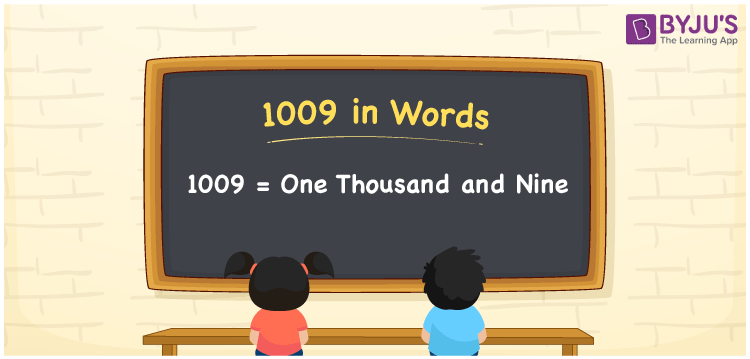# 1009 in Words

1009 in words is One thousand and nine. The number 1009 appears between 1008 and 1010. It is also a cardinal number. For example, the cost of 9 kg apples is Rs. 1009. In this article, let us learn the conversion procedure of 1009 in words using the place value system in detail.

 1009 in Words: One Thousand and Nine. One Thousand and Nine in Numerical Form: 1009.

## 1009 in English Words## How to Write 1009 in Words?

The place values of 1090 are presented in the below table.

 Thousands Hundreds Tens Ones 1 0 0 9

The expanded form of 1009 is as follows:

= 1 × Thousand + 0 × Hundred + 0 × Ten + 9 × One

= 1 × 1000 + 0 × 100 + 0 × 10 + 9 × 1

= 1000 + 9

= 1009

= One thousand and nine

Hence, 1009 in words is one thousand and nine.

1009 in words – One thousand and nine

Is 1009 an odd number? – Yes

Is 1009 an even number? – No

Is 1009 a perfect square number? – No

Is 1009 a perfect cube number? – No

Is 1009 a prime number? – Yes

Is 1009 a composite number? – No

## Frequently Asked Questions on 1009 in Words

Q1

### Write 1009 in words.

1009 in words is one thousand and nine.

Q2

### Simplify 1000 + 9, and express it in words.

Simplifying 1000 + 9, we get 1009. Hence, 1009 in words is one thousand and nine.

Q3

### Is 1009 a prime number?

Yes, 1009 is a prime number.